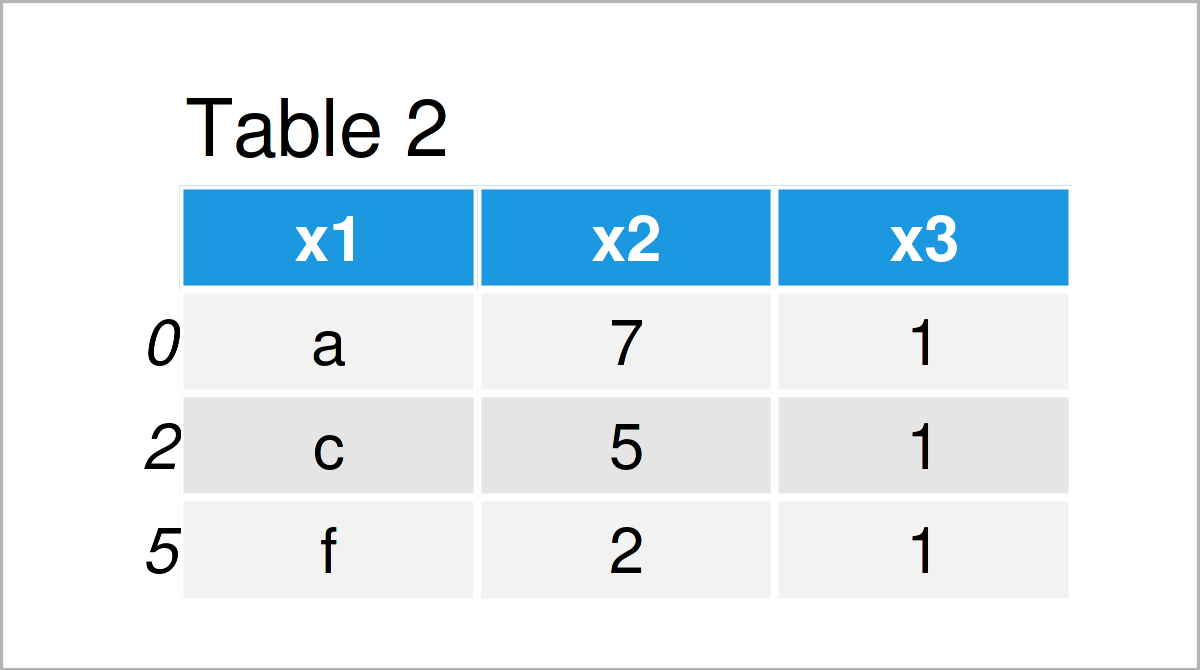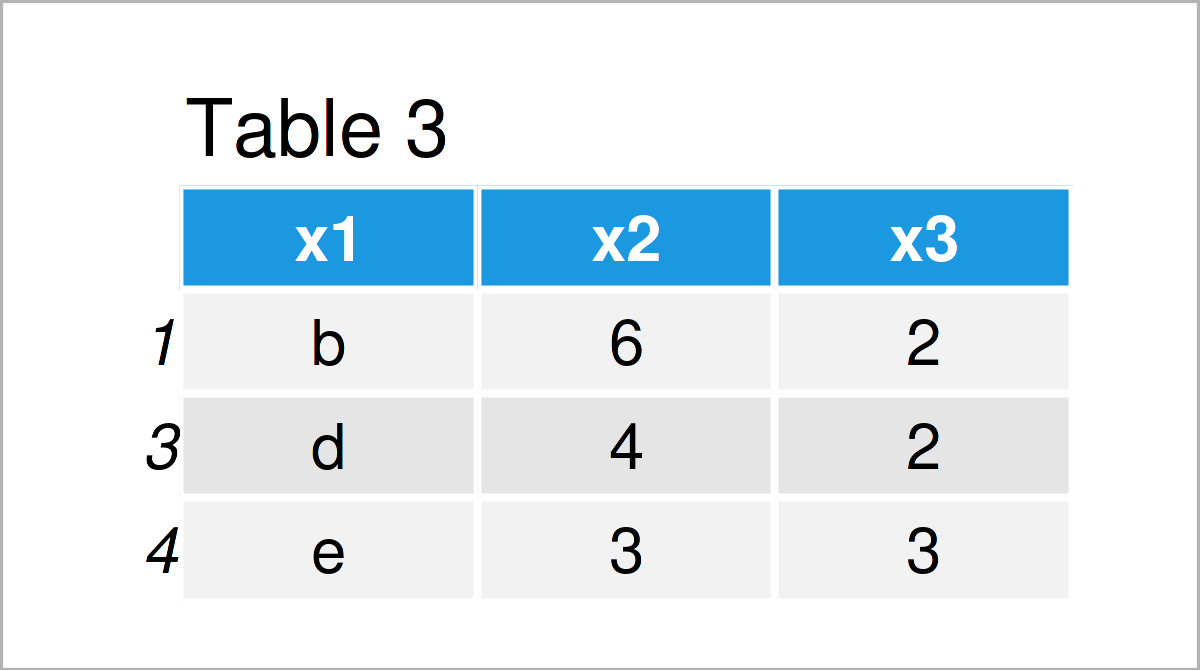# Select Rows of pandas DataFrame by Condition in Python (4 Examples)

In this article you’ll learn how to extract pandas DataFrame rows conditionally in the Python programming language.

The content of the post looks as follows:

Let’s dive right into the Python code!

## Example Data & Libraries

In order to use the functions of the pandas library, we first need to import pandas:

`import pandas as pd                                         # Import pandas`

We’ll use the following pandas DataFrame as a basis for this Python tutorial:

```data = pd.DataFrame({'x1':['a', 'b', 'c', 'd', 'e', 'f'],   # Create pandas DataFrame
'x2':range(7, 1, - 1),
'x3':[1, 2, 1, 2, 3, 1]})
print(data)                                                 # Print pandas DataFrame```Table 1 illustrates the output of the Python console and shows that our exemplifying data is made of six rows and three columns.

## Example 1: Extract Rows with Specific Value in Column

This example shows how to get rows of a pandas DataFrame that have a certain value in a column of this DataFrame.

In this specific example, we are selecting all rows where the column x3 is equal to the value 1.

We can do that as demonstrated by the Python code below:

```data_sub1 = data.loc[data['x3'] == 1]                       # Get rows with particular value
print(data_sub1)                                            # Print DataFrame subset```As shown in Table 2, the previous Python programming code has created a new pandas DataFrame called data_sub1 that contains a subset of our input data set.

## Example 2: Extract Rows with Range of Values in Column

In this example, I’ll show how to retain all lines of a pandas DataFrame where a column of this DataFrame has values in a particular range.

As in Example 1, we can use the loc attribute for this task. However, this time we have to specify a range within our logical condition:

```data_sub2 = data.loc[data['x3'] >= 2]                       # Get rows in range
print(data_sub2)                                            # Print DataFrame subset```After running the previous syntax the pandas DataFrame shown in Table 3 has been created. All rows of this DataFrame subset contain a value larger than or equal to the value 2 in the variable x3.

## Example 3: Extract Rows with Specific Set of Values in Column

The Python programming syntax below demonstrates how to access rows that contain a specific set of elements in one column of this DataFrame.

For this task, we can use the isin function as shown below:

```data_sub3 = data.loc[data['x3'].isin([1, 3])]               # Get rows with set of values
print(data_sub3)                                            # Print DataFrame subset```After running the previous syntax the pandas DataFrame shown in Table 4 has been created. This time, we have kept all rows where the column x3 contains the values 1 or 3.

## Example 4: Extract Rows Based On Multiple Columns

So far, we have specified our logical conditions only for one variable. In this example, I’ll illustrate how to search and find rows based on multiple columns.

To accomplish this, we can use the loc attribute as in Examples 1 and 2 and the & operator.

Consider the Python code below:

```data_sub4 = data.loc[(data['x2'] > 3) & (data['x3'] == 1)]  # Multiple conditions
print(data_sub4)                                            # Print DataFrame subset```By running the previous Python programming code, we have managed to create Table 5, i.e. another pandas DataFrame where we have taken a subset of particular rows based on two columns.

## Video, Further Resources & Summary

In case you need further info on the Python codes of this page, you might have a look at the following video on my YouTube channel. I explain the Python programming codes of this tutorial in the video:

Please accept YouTube cookies to play this video. By accepting you will be accessing content from YouTube, a service provided by an external third party.If you accept this notice, your choice will be saved and the page will refresh.

In addition, you may want to have a look at the other tutorials on this homepage. You can find a selection of posts below.

Summary: In this article, I have demonstrated how to get pandas DataFrame rows conditionally in Python programming. Let me know in the comments, in case you have additional questions.

Subscribe to the Statistics Globe Newsletter